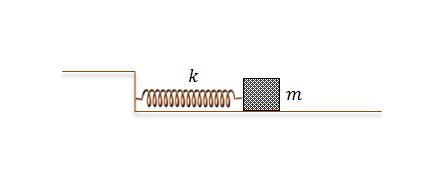Classical Mechanics

# Amplitude, Frequency, Wave Number, Phase Shift

A body of mass $m = 2\text{ kg}$ is oscillating on a spring. The position in meters of the body as a function of time $t$ can be expressed as

$x(t) = A\cos{(2\pi ft)} \text{ m} .$

If the phase of $x(t)$ is shifted by $\delta = \frac{8 \pi}{5} \text{ rad},$ the time delay of its cycle is $KT \text{ s},$ where $T$ is the period of the oscillation. What is the constant $K?$

A body of mass $m = 2\text{ kg}$ is oscillating on a spring. The position of the body in meters as a function of time $t$ can be expressed as $x(t) = A\cos{(2\pi ft)} \text{ m}.$ If $x(t)$ is delayed by $\frac{1}{3}$ of its cycle, what is the phase shift $\delta$ in radians?As illustrated in the above diagram, a body of mass $m = 4 \text{ kg}$ attached to a spring with spring constant $k = 16 \text{ N/m}$ is oscillating on a frictionless floor. Initially at $t=0,$ the position of the body was $x(t=0) = 4 \text{ m }$ and the velocity was $v(t=0) = 0 \text{ m/s }.$ What is the amplitude of the oscillation?

A body of mass $m = 2 \text{ kg}$ is oscillating on a spring. The position of the body is given as $x(t) = 4 \cos{(30 t)} \text{ m},$ where $x$ denotes the difference in the length of the spring from its original length. What is the period of the body?

Assume that $\pi = 3$.

A normal human can hear the sound frequency from $20 \text{ Hz}$ to $20000 \text{ Hz}.$ Find the longest wavelength of the hearable sound, given that the speed of sound is $340 \text{ m/s}.$

×